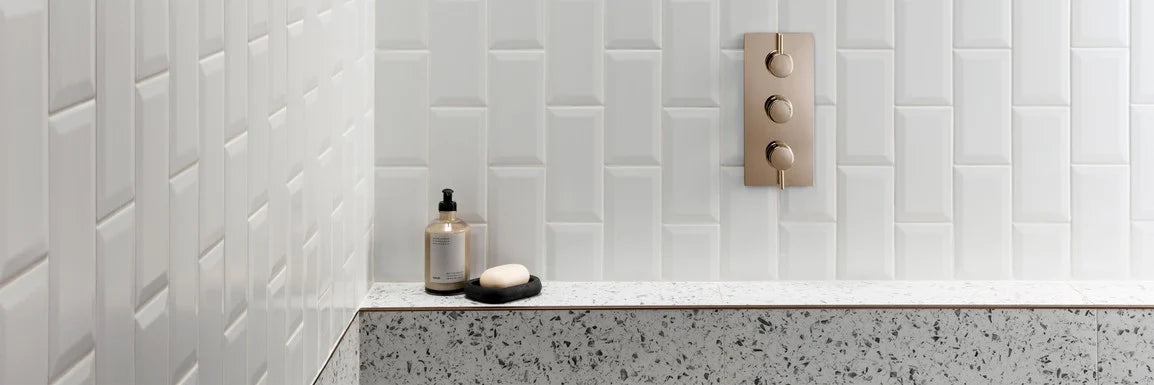## TILE CALCULATOR

If you’re planning on transforming your space with our wonderful range of floor tiles and wall tiles, be sure to use our nifty tile calculator to help you work out how many tiles you need.

Use our wall tile calculator or floor tile calculator to work out how many tiles you need for your bathrooms, kitchens and hallways. Simply enter the dimensions of your walls or floors, and let our tile calculator do all of the work for you. If you’ve got your eye on a specific tile, you can even enter the product name or the product code to get exact measurements for that tile.In order to use this tile calculator – you will first need to take measurements of your walls or your floors.

## How To Measure Walls

Step 1: Measure the length of the wall area you want to tile. Step 2: Then, measure the height of the wall area you want to tile. Step 3: Complete this for every wall you want to tile. Step 4: Using our tile calculator, input these values, using metres as a metric. Our tile calculator will calculate the area for you and show you how many tiles you need overall. Factor in your windows, doors and other obstructions using the function below.

If you already know the area of your walls – simply put the measurements into the other box below.

## How To Measure Floors

Step 1: Measure the length of the floor area you want to tile. Step 2: Then, measure the width of the floor area you want to tile. Step 3: Using our tile calculator, input these values, using metres as a metric. Our tile calculator will calculate the area for you and show you how many tiles you need overall. Factor in your windows, doors and other obstructions using the function below.

If you already know the area of your floor – simply put the measurements into the other box below.

Top Tip: Square and rectangular rooms are simple to measure – but L-shaped rooms can be a little trickier to work out. If your room is L shaped, start by roughly sketching out the shape of the floor. Then, split the sketch into two square/rectangular shapes and measure the width and length of each separate shape. Calculate the area of each shape, then, add the two areas together. This is the total area of your floor.

## How Many Tiles Do I Need?

Input your tile measurements from above to find out how many tiles you’ll need.

### Wall Tile Calculator

#### Enter the height and width of your walls below - this should be entered using metres as a metric.

Height
Width
Measurement
Wall 1
x
=
0
Wall 2
x
=
0
Wall 3
x
=
0
Wall 4
x
=
0

0
Total Wall Size: 0

### Factor in doors, windows and other obstacles

#### Enter the height and width of doors and windows below - this should be entered using metres as a metric..

Height
Width
Measurement
x
=
0
x
=
0
x
=
0
x
=
0
Total area size of doors and windows: 0

0

0 Panels

#### Need some help with your measurements?

Having trouble with finding out how many tiles you need for your walls or floors? Click below for help and advice.